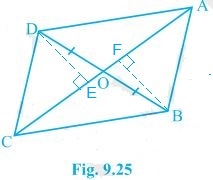Guru

# In Fig. 9.25, diagonals AC and BD of quadrilateral ABCD intersect at O such that OB = OD. If AB = CD, then show that:(ii) ar (DCB) = ar (ACB) Q.6(2)

• 0

How i solve the question of class 9th ncert math of Areas of Parallelograms and Triangles chapter of exercise 9.3of question no 6(2). I think it is very important question of class 9th give me the tricky way for solving this question In Fig. 9.25, diagonals AC and BD of quadrilateral ABCD intersect at O such that OB = OD. If AB = CD, then show that:(ii) ar (DCB) = ar (ACB)

Share

1.Given,

(ii) ar(ΔDOC) = ar(ΔAOB)

Adding ar(ΔOCB) in LHS and RHS, we get,

⇒ar(ΔDOC) + ar(ΔOCB) = ar(ΔAOB) + ar(ΔOCB)

⇒ ar(ΔDCB) = ar(ΔACB)

• 0